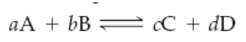×
Get Full Access to Introductory Chemistry - 5 Edition - Chapter 15 - Problem 12q
Get Full Access to Introductory Chemistry - 5 Edition - Chapter 15 - Problem 12q

×

# Solved: Write the expression for the equilibrium constant for the following genericISBN: 9780321910295 34

## Solution for problem 12Q Chapter 15

Introductory Chemistry | 5th Edition

• Textbook Solutions
• 2901 Step-by-step solutions solved by professors and subject experts
• Get 24/7 help from StudySoup virtual teaching assistantsIntroductory Chemistry | 5th Edition

4 5 1 349 Reviews
27
2
Problem 12Q

Write the expression for the equilibrium constant for the following generic chemical equation.Step-by-Step Solution:
Step 1 of 3

Solution:

The expression for the equilibrium constant for the following generic chemical equation is,The equilibrium constant is denoted as Kc

Kc = [C]c[D]d / [A]a[B]b

where:

[A], [B], [C], [D] etc. are the molar concentrations of A, B, C, D (molarity)

a, b, c, d, etc. are the coefficients in the balanced chemical equation (the numbers in front of the molecules)

Step 2 of 3

Step 3 of 3

##### ISBN: 9780321910295

Unlock Textbook Solution